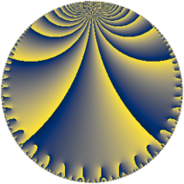# Properties

 Label 76.7.gLevel $76$ Weight $7$ Character orbit 76.g Rep. character $\chi_{76}(7,\cdot)$ Character field $\Q(\zeta_{6})$ Dimension $116$ Newform subspaces $1$ Sturm bound $70$ Trace bound $0$

# Related objects

## Defining parameters

 Level: $$N$$ $$=$$ $$76 = 2^{2} \cdot 19$$ Weight: $$k$$ $$=$$ $$7$$ Character orbit: $$[\chi]$$ $$=$$ 76.g (of order $$6$$ and degree $$2$$) Character conductor: $$\operatorname{cond}(\chi)$$ $$=$$ $$76$$ Character field: $$\Q(\zeta_{6})$$ Newform subspaces: $$1$$ Sturm bound: $$70$$ Trace bound: $$0$$

## Dimensions

The following table gives the dimensions of various subspaces of $$M_{7}(76, [\chi])$$.

Total New Old
Modular forms 124 124 0
Cusp forms 116 116 0
Eisenstein series 8 8 0

## Trace form

 $$116q - q^{2} - 67q^{4} - 2q^{5} + 33q^{6} + 962q^{8} + 12660q^{9} + O(q^{10})$$ $$116q - q^{2} - 67q^{4} - 2q^{5} + 33q^{6} + 962q^{8} + 12660q^{9} - 812q^{10} - 1462q^{12} + 2518q^{13} - 2742q^{14} + 461q^{16} + 6838q^{17} - 13948q^{18} - 24396q^{20} + 11176q^{21} + 22293q^{22} + 33905q^{24} - 156252q^{25} - 78080q^{26} - 34362q^{28} - 2q^{29} + 208344q^{30} - 10941q^{32} + 110316q^{33} + 64648q^{34} + 78574q^{36} + 55432q^{37} + 221994q^{38} + 242590q^{40} - 9126q^{41} + 187550q^{42} + 138081q^{44} + 59576q^{45} - 686052q^{46} - 141617q^{48} - 1306636q^{49} + 167498q^{50} - 35414q^{52} + 60758q^{53} - 348601q^{54} + 543732q^{56} - 466566q^{57} + 1208536q^{58} - 319142q^{60} - 839474q^{61} + 628548q^{62} - 470026q^{64} + 151836q^{65} + 1471119q^{66} + 341484q^{68} - 2169836q^{69} - 68364q^{70} + 1236042q^{72} + 207538q^{73} + 336144q^{74} - 97437q^{76} + 1977632q^{77} - 2740948q^{78} + 1250050q^{80} - 3334682q^{81} - 2244893q^{82} - 2404612q^{84} + 788426q^{85} + 910490q^{86} + 5123058q^{88} + 360166q^{89} + 2446616q^{90} + 2518692q^{92} + 2079592q^{93} - 2221200q^{94} - 5927450q^{96} + 3567778q^{97} + 5270703q^{98} + O(q^{100})$$

## Decomposition of $$S_{7}^{\mathrm{new}}(76, [\chi])$$ into newform subspaces

Label Dim. $$A$$ Field CM Traces $q$-expansion
$$a_2$$ $$a_3$$ $$a_5$$ $$a_7$$
76.7.g.a $$116$$ $$17.484$$ None $$-1$$ $$0$$ $$-2$$ $$0$$